美团面试题：String s = new String("111") 会创建几个对象？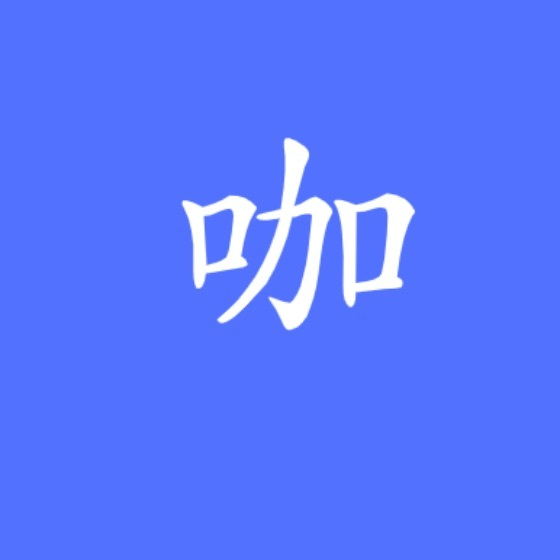Java小咖秀blog.csdn.net/o9109003234/article/details/109523691

String 不可变吗？

public class App {    public static void main(String[] args) {        String a = "111";        a = "222";        System.out.println(a);    }}

222

Stirng a="111"；

User user = new User()

String 为什么是不可变的？public final class String implements java.io.Serializable, Comparable<String>, CharSequence {    private final char value[];    public String() {        this.value = "".value;    }    public String(String original) {        this.value = original.value;        this.hash = original.hash;    }    public String(char value[]) {        this.value = Arrays.copyOf(value, value.length);    }}

String 类是 final 修饰

String 存储内容使用的是 char 数组

char 数组是 final 修饰

这里就得复习一下，final 有啥用？

/** * Description: final修饰变量 * @author : 田维常 * 欢迎关注：java后端技术全栈 */public class FinalDemo {    private final String name;
public FinalDemo(String name) { this.name = name; }
public FinalDemo() { }}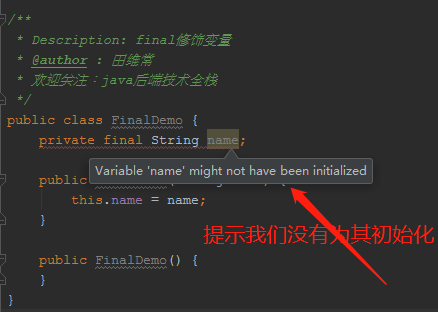/** * Description: * * @author : 田维常 * @date : 2020/11/3 * 欢迎关注公众号：java后端技术全栈 */public class StringDemo {    public static void main(String[] args) {        String name = "老田";        name.concat("!");        System.out.println(name);        System.out.println(name.concat("!"));    }}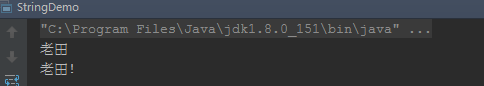public String concat(String str) {        int otherLen = str.length();        if (otherLen == 0) {            //啥都没有，就直接把当前字符串给你            return this;        }        int len = value.length;        char buf[] = Arrays.copyOf(value, len + otherLen);        str.getChars(buf, len);        //看到了吗？返回的居然是新的String对象        return new String(buf, true);    }    void getChars(char dst[], int dstBegin) {        System.arraycopy(value, 0, dst, dstBegin, value.length);    }        public String replace(char oldChar, char newChar) {        //如果两个是一样的，那就必要替换了，所以返回this        if (oldChar != newChar) {            int len = value.length;            int i = -1;            //把当前的char数组复制给val，然后下面基于val来操作            char[] val = value;
while (++i < len) { if (val[i] == oldChar) { break; } } if (i < len) { //创建一个新的char数组 char buf[] = new char[len]; for (int j = 0; j < i; j++) { buf[j] = val[j]; } while (i < len) { char c = val[i]; buf[i] = (c == oldChar) ? newChar : c; i++; } //创建一个新的String对象 return new String(buf, true); } } return this; }
public String substring(int beginIndex, int endIndex) { if (beginIndex < 0) { throw new StringIndexOutOfBoundsException(beginIndex); } if (endIndex > value.length) { throw new StringIndexOutOfBoundsException(endIndex); } int subLen = endIndex - beginIndex; if (subLen < 0) { throw new StringIndexOutOfBoundsException(subLen); } //正常返回的都是新new出来的String对象 return ((beginIndex == 0) && (endIndex == value.length)) ? this : new String(value, beginIndex, subLen); }
public String trim() { int len = value.length; int st = 0; char[] val = value; /* avoid getfield opcode */
while ((st < len) && (val[st] <= ' ')) { st++; } while ((st < len) && (val[len - 1] <= ' ')) { len--; } //如果是该字符串中包含了空格，调用substring方法，否则就是啥都没干原本返回 //就是如果字符串里有空格，那么还是新生一个String对象返回 return ((st > 0) || (len < value.length)) ? substring(st, len) : this; }

String 对象一旦被创建就是固定不变的了，对 String 对象的任何改变都不影响到原对象，相关的任何变化性的操作都会生成新的对象。

String 对象每次有变化性操作的时候，都会从新 new 一个 String 对象（这里指的是有变化的情况）。

//String a = "111";相当于char data [] ={'1','1','1'};Stirng a = new String(data);//a = "222";char data [] ={'2','2','2'};a = new String(data);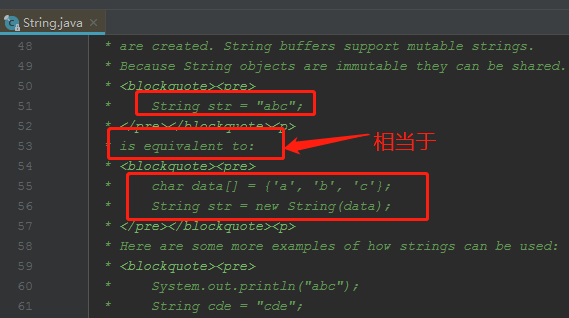public class App {    public static void main(String[] args) {        String a = "111";        String a1 = "111";
String b = new String("111");
//对象地址是同一个 System.out.println(a==a1); //对象内容是一样的 System.out.println(a.equals(a1)); //对象地址不一样 System.out.println(a==b); //对象内容是一样的 System.out.println(a.equals(b)); }}

truetruefalsetrue

a 和 a1 放在栈上，存放着对象的引用地址。

new 的对象是在堆中。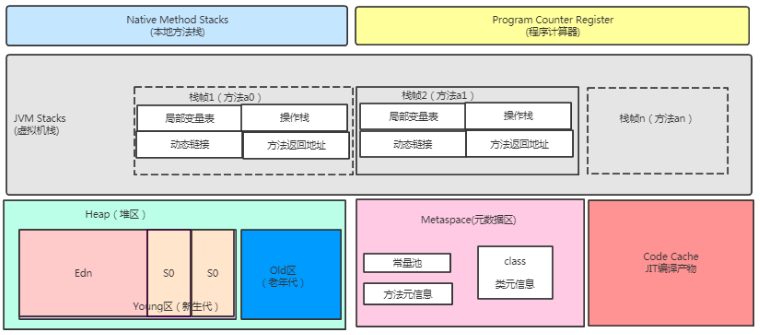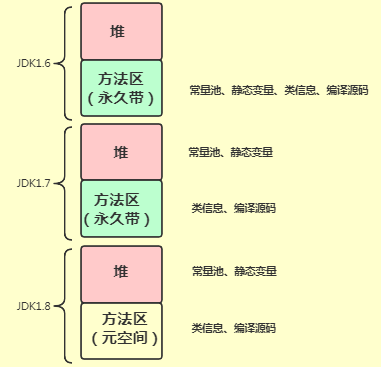评论